Courses

# Short & Long Answer Question, - Electrochemistry Class 12 Notes | EduRev

## Class 12 : Short & Long Answer Question, - Electrochemistry Class 12 Notes | EduRev

The document Short & Long Answer Question, - Electrochemistry Class 12 Notes | EduRev is a part of the Class 12 Course Chemistry for JEE.
All you need of Class 12 at this link: Class 12

Q.1 What is a Daniell cell?
Answer: Daniell cell is a device in which chemical energy converts into electrical energy. Redox reaction is responsible for the production of electricity.
Q.2 What is galvanic or voltaic cell?
Answer: The device in which chemical energy is converted into electrical energy is called a galvanic cell or electrochemical cell or voltaic cell.
Q.3 What is the difference between Galvanic Cell and Electrolytic Cell?
Answer: The metals that are used for the electronic conductance in the cell are called electrodes.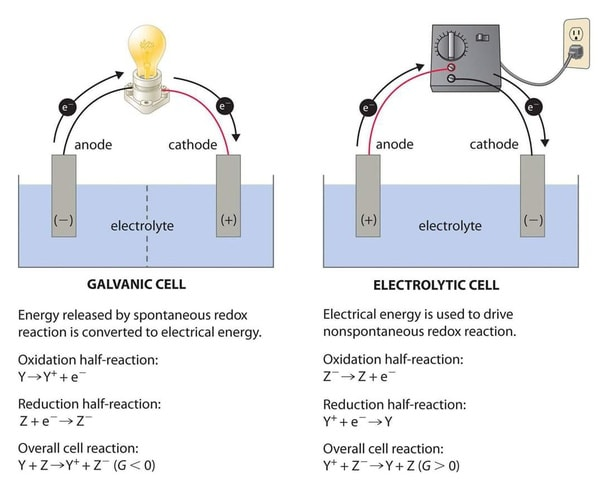Q.4 Define electrode potential.
Answer: The electrical potential difference between the metal and the solution of its ions is known as electrode potential.
Q.5 What are cathode and anode? Give their symbol.
Answer: The electrode at which oxidation occurs is the anode and that at which reduction occurs is the cathode. In an electrochemical cell, an anode electrode acts as a negative terminal and the cathode electrode acts as a positive terminal.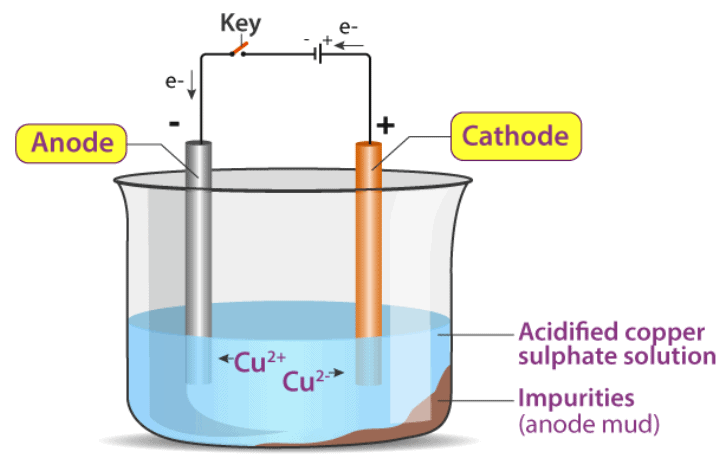Q.6 What is emf of a cell?
Answer: Cell potential is called emf of the cell when no current is drawn through the cell.
Q.7 What is the potential of S.H.E?
Answer: Zero.
Q.8 What is electrochemical series?
Answer: The arrangement of various elements in the order of increasing value of standard reduction potential is called electrochemical series.
Q.9 Write the name and polarity of an electrode where oxidation takes place in a galvanic cell.
Answer: Zn electrode.
Q.10 How does the oxidation state of manganese change in the Leclanche cell? Answer: O.S. of manganese changes from + 4 to + 3 in Leclanche cell.
Q.11 What is electrical resistance? What is its unit?
Answer: Every conducting material offers some obstruction to the flow of electricity which is called resistance. Its unit is Q, (ohm);
Q.12 What are superconductors?
Answer: The conductor which has zero resistance is called a super conductor.
Q.13 What is Faraday?
Answer: The charge transferred by 1 equivalent ion is called one Faraday    (1 F = 96500 coulomb)
Q.14 What is over potential?
Answer: Extra potential required for deposition of an ion at the electrode is called overpotential.
Q.15  Which type of reaction occurs at anode and cathode during electrolysis?Answer: Oxidation occurs at the anode and reduction occurs at the cathode during electrolysis.
Q.16 Define electrochemical equivalent.
Answer: The amount of ion deposited or liberated by one coulomb of electricity is called electrochemical equivalent.
Q.17 What is a battery?
Answer: A battery is generally a collection of Galvanic cells that is used for the production of electrical energy.
Q.18 What is a primary cell? Give example.
Answer: Primary cells are those cells that once used cannot be reused anymore. e.g. dry cell, mercury cell.
Q.19 What is a secondary cell? Give example.
Answer: The cell that can be used by recharging after it gets discharged is called a secondary cell. e.g. Lead storage battery, nickel-cadmium cell, etc.
Q.20 Which substance is used as an electrode in a dry cell?
Answer: Carbon rod as cathode, zinc cup as an anode.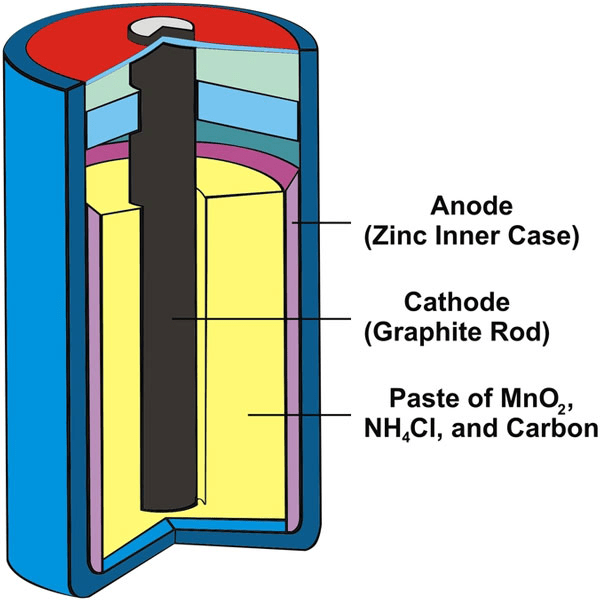Q.21 What is a Leclanche cell?
Answer: Dry cell is known as Leclanche cell.
Q.22 What is mercury cell?
Answer: Mercury cell is a primary battery that gives constant potential throughout its use.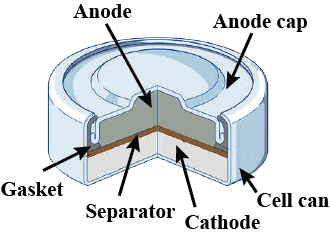Mercury CellQ.23 Name the battery used for low current devices like watches and hearing aids.
Answer: Mercury cell is used for low current devices like watches and hearing aids.
Q.24 Name the substance used as an electrolyte in a mercury cell.

Answer: Electrolyte in mercury cell is the paste of KOH and ZnO.
Q.25 The cell potential of mercury cell remains constant during their lifetime. Why?
Answer: Because cell reaction of the mercury cell does not involve. any ion. whose concentration changes. Hence it gives constant current throughout its lifetime.
Q.26 Name the battery used in automobiles and inverters.
Answer: Lead storage battery is used in automobiles and inverters.
Q.27 What are the demerits of a thermal power plant?
Answer: The demerits of the thermal power plant is that It has a very low efficiency of about 40%. It causes pollution of the atmosphere.
Q.28 What are fuel cells? Give example.
Answer: Those galvanic cells which give us direct electrical energy by the combustion of fuels like hydrogen, methane, methanol etc are called fuel cells.
Q.29 Name the fuel cell used in the Apollo space programme.
Answer: The hydrogen-oxygen fuel cell was used in the Apollo space programme.
Q.30 What is corrosion?
Answer: It is an electrochemical phenomenon in which the metal oxidises by loss of electrons in presence of water and air to form metal oxides, hydroxides or carbonates.
Q.31 Give an example of corrosion that is useful?
Answer: Formation of protective oxide layer on Al sheet.
Q.32 Name any two metals that can be used in the cathodic protection of iron.
Answer: Zinc and Mg can be used for the cathodic protection of iron.
Q.33 What is barrier protection of metal?
Answer: The processes in which iron surface is kept away from the atmosphere. By painting the surface. By coating the surface with a thin film of oil or grease. By electroplating iron with some non-corrosive metal such as nickel, chromium, copper. Using a layer of anti-rust solution e.g. b is phenol.
Q.34 Zinc can reduce hydrogen ion but copper cannot. Why?
Answer: Because in electrochemical series, standard reduction potential value of hydrogen is greater than the Zn but lower than the Cu.
Q.35 What is the role of platinum or gold as an electrode?
Answer: Platinum and gold are native metals. They are used as a support for flow of electrons.
Q.36 Explain why fluorine is the strongest oxidising agent?
Answer: Because fluorine has the highest reduction potential. (Lower electron gain enthalpy and higher solvation energy).
Q.37 Lithium metal is the strongest reducing agent. Why?
Answer: Because Li has the lowest reduction potential.
Q.38 Mention the application of electrochemical cells.
Answer: It converts chemical energy into electrical energy.
Q.39 Mention some of the applications of electrochemical series.
Answer: Higher reduction potentials are strong oxidising agents while lower reduction potentials are strong reducing agents.
(ii) The electrode with higher electrode potential (E°) acts as cathode while with lower electrode potential will act as an anode,
(iii) Predicting the feasibility of redox reaction.
(iv) Predicting the capability of a metal to displace Ha gas from acid.
Q.40 What are the factors on which electronic conductance depends?
Answer: The electronic conductance depends upon The nature and structure of the metal.The number of valence electrons per atom. Temperature (It is inversely proportional to temperature).
Q.41 On what factors conductivity of electrolytic solution depends?Answer: Electrolytic conductance depends upon the nature of the electrolyte added, the Size of ions produced and their solutions.  The nature of the solvent and its viscosity. The concentration of the electrolyte. Temperature (Directly proportional to temperature).
Q42. How is the conductivity of a solution determined?
Answer: It is determined by the use of conductivity cell using the principle of the wheat stone bridge.
Q.43 What are the difficulties in measuring the resistance of an ionic solution?Answer: 16.  There are two difficulties in measuring the resistance of an unknown solution. Passage of direct current through an ionic solution over a long period leads to change in the composition of the solution. A solution cannot be connected to the bridge like a metallic wire or any other solid conductor.
Q.44 Conductivity of a solution decreases with increasing dilution of solution. Why?
Answer: Conductivity always decreases with a decrease in concentration, because the number of ions per unit volume that carry the current in a solution decreases on dilution.
Q.45 What is an electrolytic cell?
Answer: The device in which the process of electrolysis is carried out is called electrolytic cell.
Q.46 What is electrolysis?
Answer: The process of chemical decomposition of the electrolyte by the passage of electricity through its molten or dissolved state is called electrolysis.
Q.47 Describe the construction and reactions of lead storage batteries.
Answer: The anode and cathode plates are arranged in an alternating manner and they are separated by sheets of insulating material. The anode and cathode plates are separately connected to each other. The cells are connected in series so as to increase the voltage of the battery. There are 3 cells in 6 V battery and in 12 V battery there are 6 cells.
Q.48 List important methods that can be used for the prevention of corrosion.
Answer: Important methods that can be used for the prevention of corrosion of metals are Barrier protection. (Painting, oiling, greasing, electroplating) Sacrificial protection. (Cathodic protection) Electrical protection. Use of Anti-rust solutions.
Q.49 What is sacrificial protection for corrosion?
Answer: Sacrificial protection of corrosion is an electrochemical method. In this method, metal is connected to a sacrificial electrode of another more active metal like Zn, Mg, etc which corrodes itself but saves the object.
Q.50 What is the vision of hydrogen economy?
Answer: The vision of the hydrogen economy is that hydrogen will be a renewable and non-polluting source of energy in the future.
Q.51 The blue colour of copper sulphate solution is slowly discharged when a rod of zinc is dipped in it. Explain.
Answer: Zinc lies below copper in the electrochemical series and can, therefore, release electrons to the Cu2+ ions. As a result, Zn is oxidised while Cu2+ ions are reduced. Since the concentration of Cu2+ions in the solution decreases, the blue colour of the solution is slowly discharged.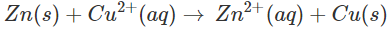Q.52 Why cannot oxidation occur without reduction?
Answer: Oxidation involves the loss of electrons while reduction involves the gain of electrons. Energy is needed when a species loses electrons and is released when it accepts electrons. This means that a species can gain energy only at the cost of electron releasing species. In other words, oxidation cannot occur without reduction or oxidation and reduction go side by side.
Q.53 Articles of iron are generally coated with zinc. Explain.
Answer: Iron gets easily rusted in the presence of moist air. The formation of rust (Fe2O3.xH2O) is a redox reaction in which Fe is oxidized to Fe3+ions. Since zinc is more reactive than iron (placed below iron in the activity series), it is oxidised to Zn2+ions instead of iron when coated over the surface of the iron. Thus, zinc takes part in the redox reaction while the surface of iron remains intact.
Q.54 Check the feasibility of the following redox reaction with the help of electrochemical series
Ni(s) + 2Ag+ (aq) → Ni2+ (aq) + 2Ag(s)
Answer: The E° value of Ni2+/Ni is? 0.25 V while that of Ag+/Ag is +0.80 V. This means that nickel is placed below silver in the series and can easily reduce Ag+ions to silver by releasing electrons. The redox reaction is, therefore, feasible.
Q.55 What will happen when chlorine is passed through an aqueous solution of potassium bromide?
Answer: The solution will acquire an orange colour due to the vapours of bromine that are evolved. Actually, chlorine is a stronger oxidising agent than bromine and oxidizes Br-ions (in KBr) to Br2 and itself is reduced to Clions.
2KBr + Cl2 → 2KCl + Br2
Q.56 What is the source of electrical energy in a galvanic cell?
Answer: In a galvanic cell, the redox reaction is of spontaneous nature. The energy as free energy (ΔG) is released in the reaction. This gets converted into electrical energy.
Q.57 How can the electrode potential of an electrode be increased?Answer: According to the Nernst equation, the electrode potential (E) of an electrode is given as: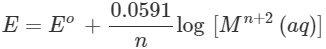The electrode potential of an electrode can be increased by increasing the concentration of the metal ions in solution. Thus, it is possible to increase the electrode potential of an electrode by adding a small amount of the same electrolyte in which the electrode is already kept.
Q.58 Knowing that: Cu2+ (aq) + 2e- → Cu(s);  E0= +0.34 V   2Ag+(aq) 2e- → 2Ag(s)-
E0 = +0⋅80V reason out whether 1M silver nitrate solution can be stored in copper vessel or 1M copper sulphate solution in a silver vessel.

Answer: A solution of an electrolyte can be stored in a particular vessel only in case there is no chemical reaction taking place with the material of the vessel. Now if silver nitrate solution is to be kept in a copper vessel, the probable reaction will be: Cu(s)+2Ag+(aq)→Cu2+(aq)+2Ag(s) Since copper is placed below silver in the activity series, this means that it is a stronger reducing agent and can lose electrons to Ag+ ions and the chemical reaction will occur. Therefore, silver nitrate is a stronger reducing agent and the solution cannot be kept in a copper vessel. Now, when copper sulphate solution is placed in a silver vessel, the likely chemical reaction is: 2Ag(s)+Cu2+(aq)→Cu(s)+2Ag+(aq) Since Ag is placed above copper in the activity series, the chemical reaction will not take place. As a result, copper sulphate can be easily stored in a silver vessel.

Q.59 In an electrochemical cell (Cu−Ag), why are solutions containing Cu2+ and Ag+ ions kept in separate containers?

Answer: In case, the two salt solutions are kept in the same container, Ag+ ions will be reduced in the same container and no external current will be generated.
Q.60 What is the difference between cell potential and standard cell potential?

Answer: A cell potential becomes standard cell potential if the temperature and pressure are 25 °C and 1 atmosphere respectively and the concentration of the electrolytes in the respective half cells is 1M.
Q.61 Write half cell reactions and balanced chemical equations for the following galvanic cells? (a) Zn(s)+|Zn2+(aq)||Cr3+(aq)|Cr(s)

(b) Pb(s),PbSO4(s)|HSO4(aq), H+(aq)||H+(aq),HSO4(aq)|PbO2(s),PbSO4(s) (c) Mg(s)|Mg2+(aq)||Sn2+(aq)|Sn(s).

Answer: (a) Zn(s)→Zn2+(aq)+2e]×3 (Oxidation half reaction)
Cr3+(aq)+3e→Cr(s)]×2 (Reduction half reaction)
= 3Zn(s)+2Cr3+(aq)→3Zn2+(aq)+2Cr(s)
(b) Pb(s)+HSO4(aq)+H2O→PbSO4(s)+H3O+(aq) (Oxidation half reaction) PbO2(s)+3H3O+(aq)+2HSO4+2e→PbSO4(s)+3H2O+2e (Reduction half reaction)
= Pb(s)+3HSO−4(aq)+PbO2(s)+2H3O+(aq)→2PbSO4(s)+4H2O.
(c) Mg(s)→Mg2+(aq)+2e  (Oxidation half reaction)
Sn2+(aq)+2e→Sn(s)  (Reduction half reaction)
=  Mg(s)+Sn2+(aq)→Mg2+(aq)+Sn(s)
Q.62 Write the cell reaction if the Nernst equation is given by the relation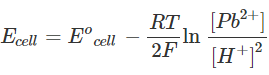Answer: The two half-cell reactions may be written as:
Pb(s)→Pb2+(aq)+2e (Oxidation half reaction)
2H+(aq)+2e→H2(g) (Reduction half reaction)
= Pb(s)+2H+(aq)→Pb2+(aq)+H2(g).
Q.63 The ionic conductance of alkali metal cations increases with an increase in atomic mass from lithium to caesium. Explain.
Answer: The alkali metal cations are monovalent (M+)Down the group, the cationic size increases and as a result, the tendency to get hydrated decreases. Since the hydration of cation decreases the ionic conductance, this means that the ionic conductance of alkali metal cations increases with an increase in atomic mass.
Q.64 Iron does not rust even if zinc coating on its surface is broken but the same is not true when the coating is of tin.

Answer: The E° values of three metals are listed as follows: Zn2+(aq)+2e→Zn(s);              Eo=−0.76V Fe2+(aq)+2e→2e(s);    Eo=−0.44V Sn2+(aq)+2e→Sn(s);    Eo=−0.14V

The data shows that zinc is more reactive than iron. This means that if a crack appears on the surface of iron coated with zinc even then zinc will take part in the redox reaction and not iron. In other words, zinc will be corroded in preference to iron. But same is not the case with tin. It is less reactive than iron. If a crack appears on the surface of iron coated with tin, then iron will take part in the redox reaction and not tin. Therefore, iron will be corroded under these circumstances.
Q.65 Rusting of iron becomes quicker in the saline medium. Explain.

Answer: Saline medium has extra salts such as sodium chloride dissolved in water. This means that it has a greater concentration of electrolyte than an ordinary medium. The ions present will favour the formation of more electrochemical cells and will thus promote rusting or corrosion.
Q.66 The mobility of H+ ions in ice is greater as compared to liquid water. Explain.

Answer: The density of liquid water is more as compared to that of ice. Therefore, ionic mobility is expected to be less in water as compared to ice. Moreover in water, the H+ ions get hydrated i.e., H+(aq). Since the hydrated ions are heavier than the H+ ions, the ionic mobility of the hydrated ions is less.
Q.67 Given that Co3++e→Co2+; Eo=+1.82V 2H2O→O2+4H++4e;Eo=−1.23V Explain why Co3+ is not stable in aqueous solution.

Answer: The Eocell can be calculated as follows:

4[Co3++e→Co2+];

Eo=+1.82V 2H2O→O2+4H++4e;  Eo=−1.23V

Add  4Co3++2H2O→4Co2++4H++O2;

Eo=+1.82−1.23=0.59V

Since Eocell is positive, the cell reaction is spontaneous. This means that the Co3+ ion will take part in the reaction. Therefore, Co3+ is not stable in an aqueous solution.
Q.68 What would happen if the protective tin coating over an iron bucket is broken in some places?

Answer: Iron will get rusted because iron has a lesser reduction potential than tin.
Q.69 Explain why zinc dissolves in dilute HCl to liberate H2(g) but from concentrated H2SO4, the gas evolved is SO2.

Answer: In dilute HCl, zinc reacts as follows: Zn+2H+(aq)→Zn2+(aq)+H2(g) Therefore, H2(g) is liberated. With concentrated H2SO4, both H+ ions and SO2−ions will be available. But SO2−4 ion is a better oxidising agent than H+ ion. Therefore, the reaction will occur as follows:
Zn(s)+SO2−4(aq)+4H+(aq)→Zn2+(aq)+SO2(g)+2H2O(aq)
Q.70 Three iron sheets have been coated separately with three metals (A, B and C) whose standard electrode potentials are given below. Metal Iron
A                  B               C                   D
-0.46V     -0.66V     -0.20V      - 0.44 V

Identify in which case rusting will take place faster when the coating is damaged.

Answer: Rusting of iron will occur faster when coated with metal C because it is placed above iron in the activity series. Iron will take part in the redox reaction and not the metal C.
Q.71 Why cannot aluminium metal be produced by the electrolysis of an aqueous solution of aluminium salt?

Answer: In an aqueous solution, H^ ions will be also formed along with Al3+ ions.H+ions will be released in preference it Al3+ ions at the cathode since their discharge potential is less. This means that Al3+ ions will remain in the solution.
Q.72 Tarnished silver contains Ag2S. Can this tarnish be removed by placing tarnished silverware in an aluminium pan containing an inert electrolyte solution such as NaCI? Given that the standard reduction potentials for the half-reactions are:
Ag2S(s)+2e→2Ag(s);+S2−(aq);Eo=−0.71V Al3+(aq)+3e→Al(s);Eo=−1.66V.

Answer: Yes, the tarnish due to silver sulphide will be removed by placing the tarnished ware in an aluminium pan. Actually, aluminium is a stronger reducing agent than silver. Therefore, it will reduce silver ions (Ag+) to metallic silver. It is silvery-white in colour will get slowly deposited on the walls of the silverware and the tarnish will be removed. Al(s)+3Ag+(aq)→Al3+(aq)+3Ag(s)

Alternatively, Eocell=Eocathode−Eoanod

e=(−0.71)−(−1.66)=0.95V   is positive.

The redox reaction is feasible and the tarnish will be removed.
Q.73 The following chemical reaction is occurring in an electrochemical cell: Mg(s)+2Ag+(0.0001M)→Mg2+(0.10M)+2Ag(s) Given EoMg2+/Mg=−2.36V;EoAg+/Ag=+0.81V For the cell, calculate/write: E° value for the electrode 2Ag+/2Ag Standard cell potential (E°) Cell potential (E) Give the symbolic representation of the above cell Will the above cell reaction be spontaneous?
Answer: (i) E° value of the electrode; 2Ag+/2Ag will remain the same i.e., 0.81 V. It does not change.
(ii) Eocell=Eocathode−Eoanode=0.81−(−2.36)=3.17V
(iii)  Ecell=Eocell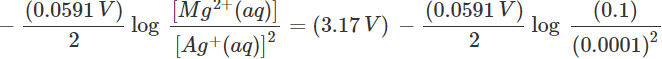=(3.17V)−(0.2068V)=2.9632V
(iv) Cell notation: Mg(s)/Mg2+(0.10M)||Ag+(0.0001M)/2Ag(s) (v) Since Mg is placed below Ag in the activity series, it is a stronger reducing agent. Therefore, the cell reaction is spontaneous.
Q.74 An electrochemical cell stops working after some time. Explain.
Answer: According to Nernst equation: E(electrode)=Eo(electrode)+0.591/n [Mn+(aq)] This means that the reduction potential of the electrode is directly proportional to the concentration of the metal ions or cations in solution. In an electrochemical cell, to start with the reduction potential of the cathode is more than that of the anode. As the redox reaction progresses in the cell, the concentration of the metal ions in the reduction half cell decreases and it increases in the oxidation half cell. Thus, the reduction potential of the anode gradually increases while that of the cathode decreases. This keeps on till the two become equal. At this stage, the cell stops working because its EMF will be zero. This has been shown graphically in the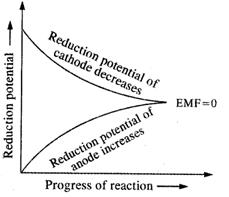Offer running on EduRev: Apply code STAYHOME200 to get INR 200 off on our premium plan EduRev Infinity!

## Chemistry for JEE

198 videos|447 docs|370 tests

,

,

,

,

,

,

,

,

,

,

,

,

,

,

,

,

,

,

,

,

,

,

,

,

;